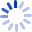# Reading and Understanding More Multivariate Statistics

This follow up volume, provides a basic understanding of certain multivariate techniques and related topics in measurement guaranteed to increase confidence in reading and understanding the statistics. Chapters demystify the use of cluster analysis, Q-technique factor analysis, structural equation modeling, canonical correlation analysis, repeated measures analyses, and survival analysis.

##### Cash for Textbooks